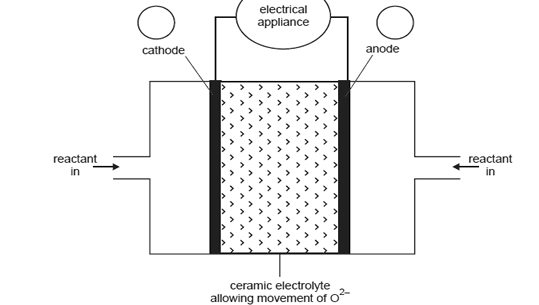Fuel cells 2006 VCE An Internet site reporting the latest developments in fuel cell technology describes a cell that uses a solid ceramic material as the electrolyte and hydrogen gas and oxygen gas as the reactants. Key features of this cell are water is the only product from the cell reaction  the ceramic material allows the movement of oxide ions (O2) the reaction at the anode is H2(g) + O2(in ceramic) → H2O(l) + 2e Operation at very high temperatures of over 1000°C means that precious metal catalysts are not required. A representation of the cell providing electricity for an appliance is shown in the diagram below.What distinguishes a fuel cell from a galvanic cell such as a dry cell or lead-acid battery? Solution On the diagram above i. in circles A and B, indicate the polarity of the cathode and anode ii. show, by using an arrow, the direction of electron flow in the external circuit. Solution Write an equation for each of the following reactions. You are not required to show states in these two equations. i. the overall cell reaction Solution ii. the reaction at the cathode Solution A ceramic fuel cell delivers a current of 0.500 A for 10.0 minutes at a potential of 0.600 volts. i. How much electrical energy, in joules, would be provided by the cell? Solution ii. Calculate the charge, in coulomb, produced by the cell. Solution iii. If this particular cell operated at 60.0% efficiency, what amount of hydrogen gas (H2), in mole, would be consumed by the fuel cell? Solution